• # 18 Search Results 'Holt Mcdougal'

• 0

Filter(s)

### All 18 results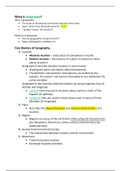#### World Geography Chapter1

(0)
\$3.49

The notes include basic informations about Geography such as Geographer's tool and the themes of geography. It also summarises about mapmaking and satellites.

•  Book
• Study guide
•  • 3 pages •
• by LilyV •
Quick View
i x
•
•#### Geography Chapter 1 Looking at the Earth Notes Outline pt 1

(0)
\$7.49

Geography notes in outline format. Chapter 1 Looking at the Earth notes. Covers the 5 themes of geography, absolute and relative location, latitude and longitude, formal, functional, and perceptual regions, linear and time distance, and psychological distance.

•  Book
• Class notes
•  • 0 pages •
Quick View
i x
•
•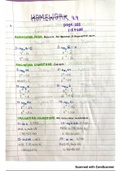#### Evaluate Logarithms and Graph Logarithmic Functions

(0)
\$7.49

This document includes homework assignments from the "Holt McDougal Larson Algebra 2" textbook. It focuses on how to rewrite logarithms, evaluate logarithms, calculate logarithms with and without a calculator, using inverse properties, finding the inverse, and graphing functions. There are multiple examples included for each exercise, as well as their answers, calculations, and graphs.

•  Bundle
• Textbook notes
•  • 3 pages •
• by biancacirigliano •
Quick View
i x
•
•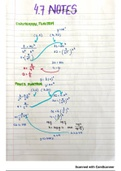#### Exponential and Power Functions

(0)
\$7.49

These are class notes taken for "Exponential and Logarithmic Functions", which is chapter 4 on the "Holt McDougal Larson Algebra 2" textbook. These are colorful notes including step-by-step solving, as well as examples and practice problems. Each mathematical equation is also written down according to the section and its problems to solve. This focuses specifically on exponential functions and power functions. Throughout the notes, each section is labeled with a colored title that helps foll...

•  Book & Bundle
• Class notes
•  • 1 pages •
• by biancacirigliano •
Quick View
i x
•
•
•#### Sell your knowledge on Stuvia

Hundred thousands of people are searching for your content every day. You can easily upload your summaries to our platform and start earning money from your study notes.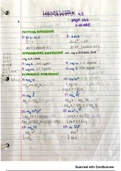#### Apply Properties of Logarithms

(0)
\$7.49

These are homework problems from the "Holt McDougal Larson Algebra 2" textbook, chapter 4 "Exponential and Logarithmic Functions", lesson 4.5 "Apply Properties of Logarithms". These example problems focus mainly on expressions. This includes matching expressions, approximating expressions, expanding expressions, condensing expressions, and applying the change of base formula. This document is separated into sections, titled according to the exercise, along with directions, as well as their...

•  Book & Bundle
• Textbook notes
•  • 2 pages •
• by biancacirigliano •
Quick View
i x
•
•
•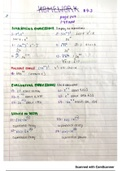#### Evaluate Logarithms and Graph Logarithmic Functions

(0)
\$7.49

These are homework problems from the "Holt McDougal Larson Algebra 2" textbook, chapter 4 "Exponential and Logarithmic Functions", lesson 4.4 "Evaluate Logarithms and Graph Logarithmic Functions". These example problems focus mainly on logarithms. This includes exponential forms, rewriting equations, evaluating logarithms, calculating logarithms with and without a calculator, using inverse properties, finding inverses, and graphing functions. This document is separated into sections, title...

•  Book & Bundle
• Textbook notes
•  • 2 pages •
• by biancacirigliano •
Quick View
i x
•
•
•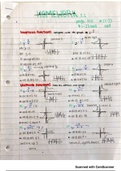#### Graph Simple Rational Functions

(0)
\$7.49

These are homework problems from the "Holt McDougal Larson Algebra 2" textbook, chapter 5 "Rational Functions", lesson 5.2 "Graph Simple Rational Functions". These example problems focus mainly on graphing functions. This includes comparing to a set graph, stating domain and range, finding asymptotes and graphing. All graphs, asymptotes, domain and range are shown for each one of the exercises. This document is separated into sections, titled according to the exercise, along with direction...

•  Book & Bundle
• Textbook notes
•  • 2 pages •
• by biancacirigliano •
Quick View
i x
•
•
•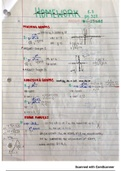#### Graph General Rational Functions

(0)
\$7.49

These are homework problems from the "Holt McDougal Larson Algebra 2" textbook, chapter 5 "Rational Functions", lesson 5.3 "Graph General Rational Functions". These example problems focus mainly on graphing rational functions. This includes matching graphs, analyzing graphs, identifying asymptotes, identifying x interceptx-interc, and graphing the function. All x-intercepts, horizontal asymptotes (HA), and vertical asymptotes (VA) are shown. This document is separated into sections, titled...

•  Book & Bundle
• Textbook notes
•  • 2 pages •
• by biancacirigliano •
Quick View
i x
•
•
•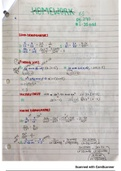#### Add and Subtract Rational Expressions

(0)
\$7.49

These are homework problems from the "Holt McDougal Larson Algebra 2" textbook, chapter 5 "Rational Functions", lesson 5.5 "Add and Subtract Rational Expressions". These example problems focus mainly on rational expressions. This includes linking denominators, finding least common denominators (LCMS), unlike denominators, and simplifying complex fractions. This document is separated into sections, titled according to the exercise, along with directions, as well as their answers, in order t...